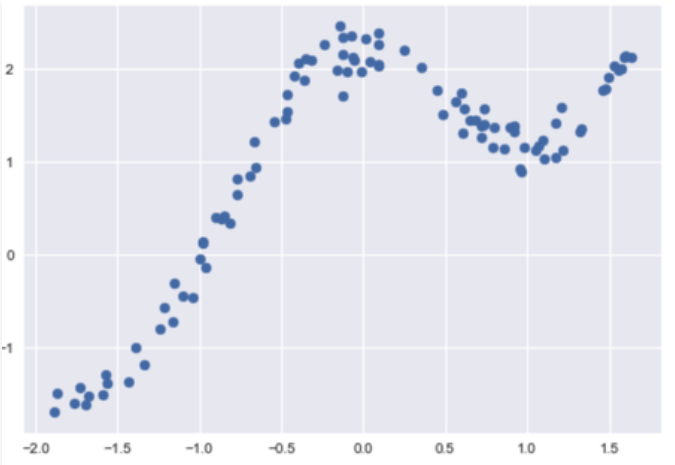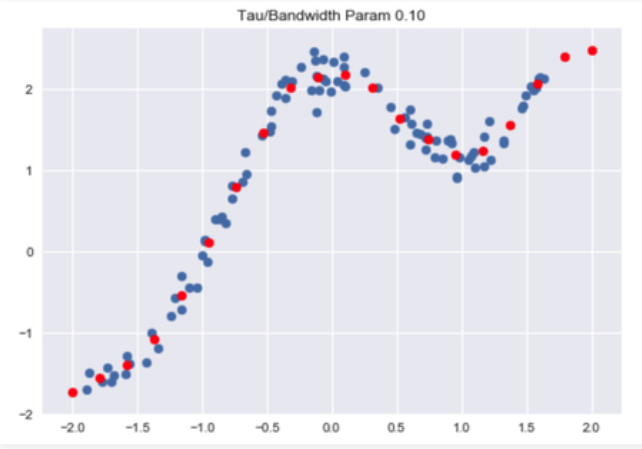# Implementation of Locally Weighted Linear Regression

• Difficulty Level : Expert
• Last Updated : 12 Dec, 2021

LOESS or LOWESS are non-parametric regression methods that combine multiple regression models in a k-nearest-neighbor-based meta-model. LOESS combines much of the simplicity of linear least squares regression with the flexibility of nonlinear regression. It does this by fitting simple models to localized subsets of the data to build up a function that describes the variation in the data, point by point.

• This algorithm is used for making predictions when there exists a non-linear relationship between the features.
• Locally weighted linear regression is a supervised learning algorithm.
• It a non-parametric algorithm.
• doneThere exists No training phase. All the work is done during the testing phase/while making predictions.

Suppose we want to evaluate the hypothesis function h at a certain query point x. For linear regression we would do the following:For locally weighted linear regression we will instead do the following:where w(i) is a is a non-negative “weight” associated with training point x(i). A higher “preference” is given to the points in the training set lying in the vicinity of x than the points lying far away from x. so For x(i) lying closer to the query point x, the value of w(i) is large, while for x(i) lying far away from x the value of w(i) is small.
w(i) can be chosen as –Directly using closed Form solution to find parameters-Code: Importing Libraries :

## python

 import numpy as npimport matplotlib.pyplot as pltimport pandas as pd plt.style.use("seaborn")

## python

 # Loading CSV files from local storagedfx = pd.read_csv('weightedX_LOWES.csv')dfy = pd.read_csv('weightedY_LOWES.csv')# Getting data from DataFrame Object and storing in numpy n-dim arraysX = dfx.valuesY = dfy.values

Output:Code: Function to calculate weight matrix :

## python

 # function to calculate W weight diagonal Matrix used in calculation of predictionsdef get_WeightMatrix_for_LOWES(query_point, Training_examples, Bandwidth):  # M is the No of training examples  M = Training_examples.shape  # Initialising W with identity matrix  W = np.mat(np.eye(M))  # calculating weights for query points  for i in range(M):    xi = Training_examples[i]    denominator = (-2 * Bandwidth * Bandwidth)    W[i, i] = np.exp(np.dot((xi-query_point), (xi-query_point).T)/denominator)    return W

Code: Making Predictions:

## python

 # function to make predictionsdef predict(training_examples, Y, query_x, Bandwidth):  M = Training_examples.shape  all_ones = np.ones((M, 1))  X_ = np.hstack((training_examples, all_ones))  qx = np.mat([query_x, 1])  W = get_WeightMatrix_for_LOWES(qx, X_, Bandwidth)  # calculating parameter theta  theta = np.linalg.pinv(X_.T*(W * X_))*(X_.T*(W * Y))  # calculating predictions  pred = np.dot(qx, theta)  return theta, pred

Code: Visualise Predictions :

## python

 # visualise predicted values with respect# to original target values Bandwidth = 0.1X_test = np.linspace(-2, 2, 20)Y_test = []for query in X_test:  theta, pred = predict(X, Y, query, Bandwidth)  Y_test.append(pred)horizontal_axis = np.array(X)vertical_axis = np.array(Y)plt.title("Tau / Bandwidth Param %.2f"% Bandwidth)plt.scatter(horizontal_axis, vertical_axis)Y_test = np.array(Y_test)plt.scatter(X_test, Y_test, color ='red')plt.show()My Personal Notes arrow_drop_up